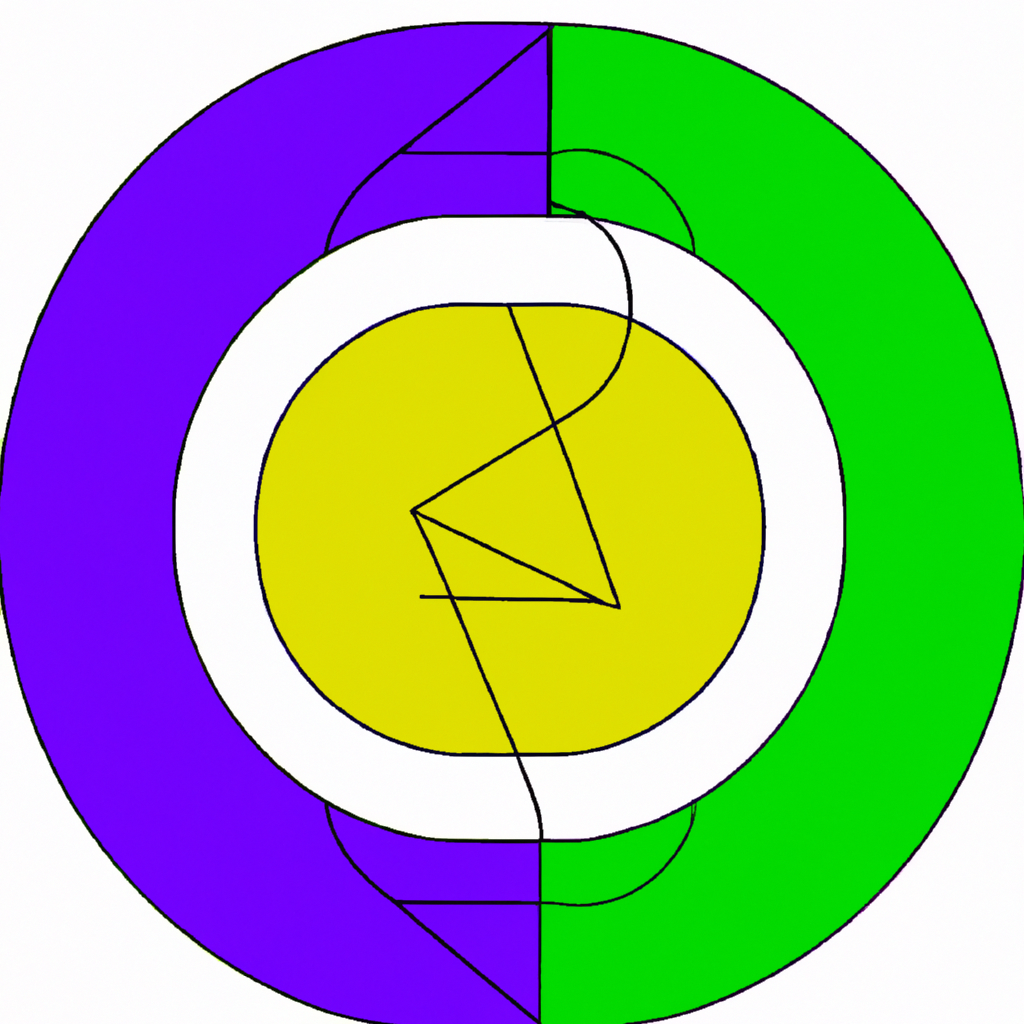Python

# Understanding the Python len() Function: A Comprehensive Guide

In this tutorial, we will delve into one of Python’s built-in functions, the `len()` function. This function is a simple yet powerful tool that can be used to determine the length (the number of items) of an object.

## What is the len() Function?

The `len()` function in Python is used to find out how many items are present in a given object. The object could be a list, tuple, dictionary, string, or any other iterable.

``````
# Example usage
my_list = [1, 2, 3]
print(len(my_list)) # Output: 3
```
```

## Syntax of len() Function

The syntax for using the `len()` function is quite straightforward:

``````
len(object)
```
```

The ‘object’ parameter represents the iterable whose length you want to find out.

## Detailed Usage of len() Function

### Using len() with Lists and Tuples

You can use the `len()` function with lists and tuples as follows:

``````
# Using len() with lists
my_list = [1, 2, 'Python', 'Tutorial']
print(len(my_list)) # Output: 4

# Using len() with tuples
my_tuple = ('Python', 'is', 'awesome')
print(len(my_tuple)) # Output: 3
```
```

### Using len() with Dictionaries and Sets

You can also use it with dictionaries and sets:

``````
# Using len() with dictionaries
my_dict = {'name': 'John', 'age': 25, 'country': 'USA'}
print(len(my_dict)) # Output: 3

# Using len() with sets
my_set = {1, 2, 3, 4}
print(len(my_set)) # Output: 4
```
```

### Using len() with Strings

When used with strings, the `len()` function returns the number of characters in the string.

``````
# Using len() with strings
my_string = "Python"
print(len(my_string)) # Output: 6
```
```

## Conclusion

The Python `len()` function is a versatile tool that can help you determine the length or count of items in various iterable objects. It’s simplicity and wide applicability make it an essential part of any Python programmer’s toolkit.

We hope this tutorial has helped you understand how to use the `len()` function effectively. Happy coding!